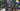# Calculating Expected Value

By Evan JarvisFriday Mar 05, 2021## Lesson #3: How To Calculate Expected Value

Calculating the expected value (EV) is at the heart of every decision you make in poker. Not every poker superstar knows EV equations, but they do grasp the theory of it. For those of you that can pick up math easily, or are willing to learn, if you can wrap your head around EV then you’ll improve your decision making and your game.

## What’s Expected Value?

Here’s why you should you care about expected value:

1. EV allows you to determine if a play is profitable or unprofitable, and to what degree.
2. EV compares the values of various strategic options (betting, folding).

If you want to become a better poker player, pick up EV and incorporate it into your game so you can make the most profitable decisions. And grab a copy of The Cash Game Guide for even more techniques to improve your game.

## How to Calculate Expected Value (EV)

• Expected Value = [value of outcome][odds of outcome]
• Inside the Brackets: Calculate these first
• Brackets Side-by-side: Multiply these values

To calculate EV, you put values into brackets and tally the results. The values are the expected gain or loss, multiplied by the odds or frequency of that happening (how often will you win or lose). Put it together, and boom you have your EV of a situation.

• If something happens 100% of the time, EV is the value of the outcome.
• If something never happens, anything multiplied by zero will always be zero.
• If an event will happen some percentage of the time, and another event will happen some other percentage of the time, that’s when we do some multiplication and addition to get the total value.

## Folding

#### EV(Fold) = 0

Whenever you fold, you’re surrendering the pot and stand no chance of winning or gaining any value. Even if you’ve contributed to the pot, that money is no longer yours, it belongs to the pot. What happens leading up to your folding, doesn’t matter. So when calculating the EV of a fold: the value of 0 x the frequency of 100 is 0.

## Calling

EV(Call) = [pot+bet]*[call and win] – [bet]*[call and lose]

The EV of a call is how much you stand to win or lose if you call. Let’s simplify: the EV of a call is the pot size plus their bet multiplied by the odds of you calling and winning, subtracted by their bet multiplied by the odds of you calling and losing.

#### If drawing: calculate equity by counting your outs

To determine your equity when drawing, count your outs and look at the cards remaining. Your odds of losing are the inverse.

#### If ahead: calculate equity by counting brick cards

If you have the best hand your odds of losing are your opponent’s chances of winning—how many outs do they have? Your odds of winning are how many brick cards can come on the river; how many give you the winning or losing hand? But if you’re on the river, your odds of calling and winning are based on how many hands in your opponents range you can beat, and how many hands have you beat. These are the odds for second and fourth boxes.

The key thing to remember when looking at how much you stand to win, is both your opponents bet and how much the pot is worth. On the flipside, all you will lose if you call is another bet. Until the pot is claimed it belongs to no one; therefore, you can’t lose the value of the bet.

## Betting

#### EV(Bet) = [pot]*[fold frequency]+[pot+bet]*[opponent calls and loses] – [bet]*[opponent calls and wins]

The final element of EV, is the value you gain by making him fold. When you first start calculating fold frequency, until you get the hang of it write it out. First look at the pot size, then figure out your opponents fold frequency. To calculate their fold frequency map out their range (fold, lose, win) by assigning them values and putting them into boxes.

## Calculating Expected Value

If you want to increase your bankroll and make better choices while you’re at the felt, learning expected value is your secret to success. EV isn’t rocket science, but it will take some getting used too, so practice after each session until it becomes second nature.